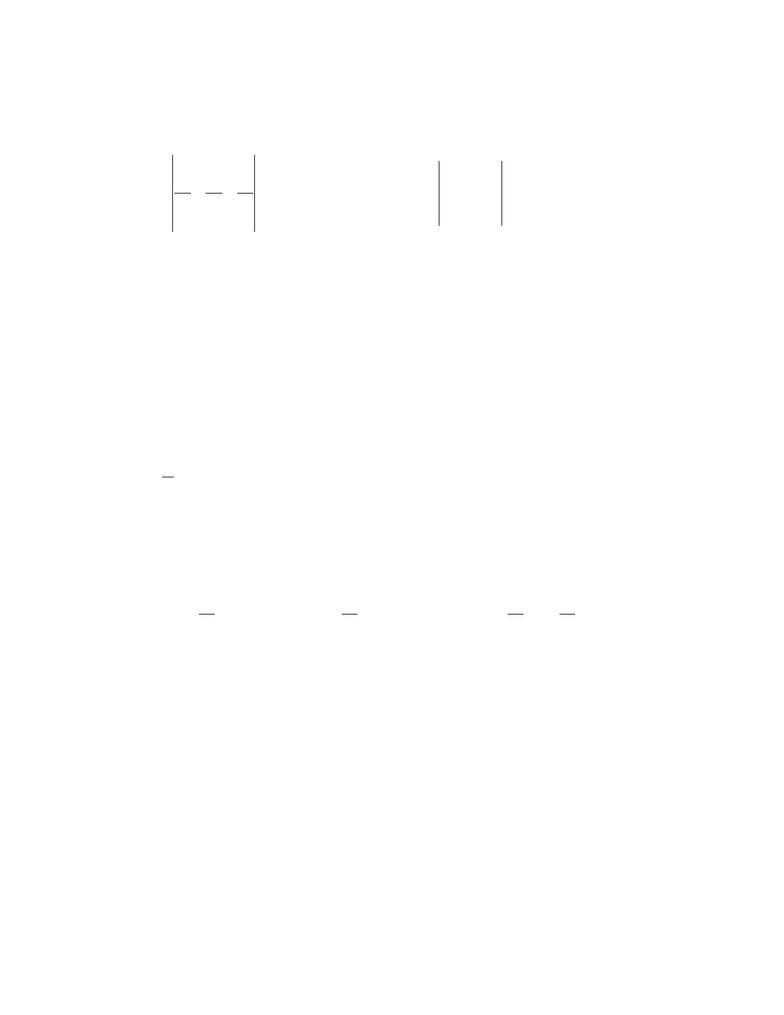Study Guides (390,000)
CA (150,000)
UTSG (10,000)
MAT (1,000)
all (20)

# MAT237Y1 Study Guide - Piecewise, Density

This preview shows page 1. to view the full 4 pages of the document.1. Suppose where a is a constant. kjiF yxazzyx ++=),,(
(a) [5 marks] Is there a real number a such that 0])[( >
×
FFcurldiv . Justify.
We have
)1,,1( a
yxaz zyx
curl =
=
kji
F and
=
×
FF)(curl ),,(11 2zaxyazxay
yxaz
a=
kji
Hence for all values of a and so there is no value of a 011])[( 2<=× acurldiv FF
such that . 0])[( >× FFcurldiv
(b) [7 marks] In case , evaluate , where C is the portion of the curve of
2=a
C
dxF
intersection of surfaces and in the first octant from o
2
xz =4
22 =+ yx )4,0,2( t )0,2,0(.
In this case kjiF yxzzyx
+
+= 2),,(. From and (both equations should
be satisfied) we get a required parametrization of C :
222 2=+ yx
x2
2
xz =
tcos
=
, tsiny2
=
, , tz 2
cos4=
2
0
π
t and we have
C
dxF = =
2/
0
2)sincos8,cos2,sin2()sin2,cos2,cos8(
π
dtttttttt
= =
+
2/
0
222 )cossin16cos4sincos16(
π
dtttttt
=
ππ
π
=++++=++)000
3
16
()
3
16
00(]sin
3
16
2sin2cos
3
16
[2/
0
33 tttt
2